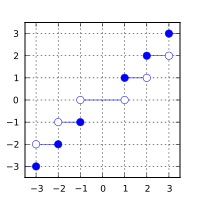/C

# trunc, truncf, truncl

Defined in header `<math.h>`
`float       truncf( float arg );`
(1) (since C99)
`double      trunc( double arg );`
(2) (since C99)
`long double truncl( long double arg );`
(3) (since C99)
Defined in header `<tgmath.h>`
`#define trunc( arg )`
(4) (since C99)
1-3) Computes the nearest integer not greater in magnitude than `arg`.
4) Type-generic macro: If `arg` has type `long double`, `truncl` is called. Otherwise, if `arg` has integer type or the type `double`, `trunc` is called. Otherwise, `truncf` is called.

### Parameters

 arg - floating point value

### Return value

If no errors occur, the nearest integer value not greater in magnitude than `arg` (in other words, `arg` rounded towards zero), is returned.

Return valueArgument

### Error handling

Errors are reported as specified in math_errhandling.

If the implementation supports IEEE floating-point arithmetic (IEC 60559),

• The current rounding mode has no effect.
• If `arg` is ±∞, it is returned, unmodified
• If `arg` is ±0, it is returned, unmodified
• If arg is NaN, NaN is returned

`FE_INEXACT` may be (but isn't required to be) raised when truncating a non-integer finite value.

The largest representable floating-point values are exact integers in all standard floating-point formats, so this function never overflows on its own; however the result may overflow any integer type (including `intmax_t`), when stored in an integer variable.

The implicit conversion from floating-point to integral types also rounds towards zero, but is limited to the values that can be represented by the target type.

### Example

```#include <math.h>
#include <stdio.h>
int main(void)
{
printf("trunc(+2.7) = %+.1f\n", trunc(2.7));
printf("trunc(-2.7) = %+.1f\n", trunc(-2.7));
printf("trunc(-0.0) = %+.1f\n", trunc(-0.0));
printf("trunc(-Inf) = %+f\n",   trunc(-INFINITY));
}```

Possible output:

```trunc(+2.7) = +2.0
trunc(-2.7) = -2.0
trunc(-0.0) = -0.0
trunc(-Inf) = -inf```
• C11 standard (ISO/IEC 9899:2011):
• 7.12.9.8 The trunc functions (p: 253-254)
• 7.25 Type-generic math <tgmath.h> (p: 373-375)
• F.10.6.8 The trunc functions (p: 528)
• C99 standard (ISO/IEC 9899:1999):
• 7.12.9.8 The trunc functions (p: 234)
• 7.22 Type-generic math <tgmath.h> (p: 335-337)
• F.9.6.8 The trunc functions (p: 464)

### See also

 floorfloorffloorl (C99)(C99) computes largest integer not greater than the given value (function) ceilceilfceill (C99)(C99) computes smallest integer not less than the given value (function) roundlroundllround (C99)(C99)(C99) rounds to nearest integer, rounding away from zero in halfway cases (function)

© cppreference.com
Licensed under the Creative Commons Attribution-ShareAlike Unported License v3.0.
http://en.cppreference.com/w/c/numeric/math/trunc